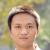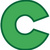## JFinal 调用yourMode.save()，遇到关键字怎么处理？

this.set("[index]", index);

0this.put(key, value) 即可打完收工0@JFinal 如下代码改写，自动处理SQLServer关键字。

```package com.rocbin.jfinal.dialect;

import java.util.List;
import java.util.Map;
import java.util.Map.Entry;
import java.util.Set;

import com.jfinal.plugin.activerecord.Record;
import com.jfinal.plugin.activerecord.Table;
import com.jfinal.plugin.activerecord.dialect.AnsiSqlDialect;

/**
* AnsiSqlDialect 扩展，自动SQL关键字作为列名时的语法冲突
*
* @author Rocbin 2015
* @version 1.0
*
*/
public class SQLServerDialect extends AnsiSqlDialect {

private static final String SQL_WORDS_STRING = "ADD,EXTERNAL,PROCEDURE,ALL,FETCH,"
+ "PUBLIC,ALTER,FILE,RAISERROR,AND,FILLFACTOR,READ,ANY,"
+ "FOR,READTEXT,AS,FOREIGN,RECONFIGURE,ASC,FREETEXT,"
+ "REFERENCES,AUTHORIZATION,FREETEXTTABLE,REPLICATION,"
+ "BACKUP,RESTORE,BEGIN,FULL,RESTRICT,BETWEEN,FUNCTION,"
+ "RETURN,BREAK,GOTO,REVERT,BROWSE,GRANT,REVOKE,BULK,GROUP"
+ ",RIGHT,BY,HAVING,ROLLBACK,CASCADE,HOLDLOCK,ROWCOUNT,CASE,"
+ "IDENTITY,ROWGUIDCOL,CHECK,IDENTITY_INSERT,RULE,CHECKPOINT,"
+ "IDENTITYCOL,SAVE,CLOSE,IF,SCHEMA,CLUSTERED,IN,SECURITYAUDIT,"
+ "COALESCE,INDEX,SELECT,COLLATE,INNER,SEMANTICKEYPHRASETABLE,"
+ "COLUMN,INSERT,SEMANTICSIMILARITYDETAILSTABLE,COMMIT,INTERSECT,"
+ "SEMANTICSIMILARITYTABLE,COMPUTE,INTO,SESSION_USER,CONSTRAINT,"
+ "is,SET,CONTAINS,JOIN,SETUSER,CONTAINSTABLE,KEY,SHUTDOWN,CONTINUE,"
+ "KILL,SOME,CONVERT,LEFT,STATISTICS,CREATE,LIKE,SYSTEM_USER,CROSS,"
+ "LINENO,TABLE,CURRENT,LOAD,TABLESAMPLE,CURRENT_DATE,MERGE,TEXTSIZE,"
+ "CURRENT_TIME,NATIONAL,THEN,CURRENT_TIMESTAMP,NOCHECK,CURRENT_USER,"
+ "NONCLUSTERED,TOP,CURSOR,NOT,TRAN,DATABASE,NULL,TRANSACTION,DBCC,NULLIF,"
+ "Trigger,DEALLOCATE,OF,TRUNCATE,DECLARE,OFF,TRY_CONVERT,DEFAULT,OFFSETS,"
+ "TSEQUAL,DELETE,ON,UNION,DENY,OPEN,UNIQUE,DESC,OPENDATASOURCE,UNPIVOT,DISK,"
+ "OPENQUERY,UPDATE,DISTINCT,OPENROWSET,UPDATETEXT,DISTRIBUTED,OPENXML,USE,DOUBLE,"
+ "OPTION,USER,DROP,OR,VALUES,DUMP,ORDER,VARYING,ELSE,OUTER,View,END,OVER,WAITFOR,"
+ "ERRLVL,PERCENT,WHEN,ESCAPE,PIVOT,WHERE,EXCEPT,PLAN,WHILE,EXEC,PRECISION,WITH,"
+ "EXECUTE,PRIMARY,WITHIN GROUP,EXISTS,PRINT,WRITETEXT,EXIT,PROC";
private static final String[] SQL_WORDS = SQL_WORDS_STRING.split(",");

@Override
public String forTableBuilderDoBuild(String tableName) {
return "select * from " + tableName + " where 1 = 2";
}

@Override
public void forModelSave(Table table, Map<String, Object> attrs,
StringBuilder sql, List<Object> paras) {
sql.append("insert into ").append(table.getName()).append("(");
StringBuilder temp = new StringBuilder(") values(");
for (Entry<String, Object> e : attrs.entrySet()) {
String colName = e.getKey();
if (table.hasColumnLabel(colName)) {
if (paras.size() > 0) {
sql.append(", ");
temp.append(", ");
}
sql.append(handlerColName(colName));
temp.append("?");
paras.add(e.getValue());
}
}
sql.append(temp.toString()).append(")");
}

@Override
public String forModelDeleteById(Table table) {
String pKey = table.getPrimaryKey();
StringBuilder sql = new StringBuilder(45);
sql.append("delete from ");
sql.append(table.getName());
sql.append(" where ").append(pKey).append(" = ?");
return sql.toString();
}

@Override
public void forModelUpdate(Table table, Map<String, Object> attrs,
Set<String> modifyFlag, String pKey, Object id, StringBuilder sql,
List<Object> paras) {
sql.append("update ").append(table.getName()).append(" set ");
for (Entry<String, Object> e : attrs.entrySet()) {
String colName = e.getKey();
if (!pKey.equalsIgnoreCase(colName) && modifyFlag.contains(colName)
&& table.hasColumnLabel(colName)) {
if (paras.size() > 0)
sql.append(", ");
sql.append(handlerColName(colName)).append(" = ? ");
paras.add(e.getValue());
}
}
sql.append(" where ").append(pKey).append(" = ?");
paras.add(id);
}

@Override
public String forModelFindById(Table table, String columns) {
StringBuilder sql = new StringBuilder("select ");
if (columns.trim().equals("*")) {
sql.append(columns);
} else {
String[] columnsArray = columns.split(",");
for (int i = 0; i < columnsArray.length; i++) {
if (i > 0)
sql.append(", ");
sql.append(columnsArray[i].trim());
}
}
sql.append(" from ");
sql.append(table.getName());
sql.append(" where ").append(table.getPrimaryKey()).append(" = ?");
return sql.toString();
}

@Override
public String forDbFindById(String tableName, String primaryKey,
String columns) {
StringBuilder sql = new StringBuilder("select ");
if (columns.trim().equals("*")) {
sql.append(columns);
} else {
String[] columnsArray = columns.split(",");
for (int i = 0; i < columnsArray.length; i++) {
if (i > 0)
sql.append(", ");
sql.append(columnsArray[i].trim());
}
}
sql.append(" from ");
sql.append(tableName.trim());
sql.append(" where ").append(primaryKey).append(" = ?");
return sql.toString();
}

@Override
public String forDbDeleteById(String tableName, String primaryKey) {
StringBuilder sql = new StringBuilder("delete from ");
sql.append(tableName.trim());
sql.append(" where ").append(primaryKey).append(" = ?");
return sql.toString();
}

@Override
public void forDbSave(StringBuilder sql, List<Object> paras,
String tableName, Record record) {
sql.append("insert into ");
sql.append(tableName.trim()).append("(");
StringBuilder temp = new StringBuilder();
temp.append(") values(");

for (Entry<String, Object> e : record.getColumns().entrySet()) {
if (paras.size() > 0) {
sql.append(", ");
temp.append(", ");
}
sql.append(handlerColName(e.getKey()));
temp.append("?");
paras.add(e.getValue());
}
sql.append(temp.toString()).append(")");
}

@Override
public void forDbUpdate(String tableName, String primaryKey, Object id,
Record record, StringBuilder sql, List<Object> paras) {
sql.append("update ").append(tableName.trim()).append(" set ");
for (Entry<String, Object> e : record.getColumns().entrySet()) {
String colName = e.getKey();
if (!primaryKey.equalsIgnoreCase(colName)) {
if (paras.size() > 0) {
sql.append(", ");
}
sql.append(handlerColName(colName)).append(" = ? ");
paras.add(e.getValue());
}
}
sql.append(" where ").append(primaryKey).append(" = ?");
paras.add(id);
}

/**
* 处理列名，如果是关键字则返回'[' + 列名 + ']'
*
* @param name
* @return
*/
public String handlerColName(String name) {
boolean isSqlWord = false;
for (int i = 0; i < SQL_WORDS.length; i++) {
if (name.equalsIgnoreCase(SQL_WORDS[i])) {
isSqlWord = true;
break;
}
}
if (isSqlWord) {
return "[".concat(name).concat("]");
} else {
return name;
}
}
}```